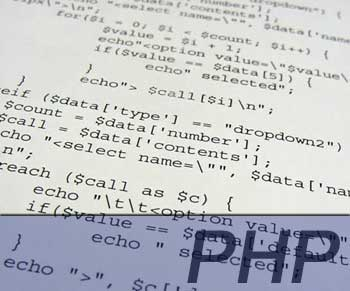# 用while next訪問陣列，比foreach快上近7倍？******************************************************
30870 Element Array Traversing
[test_time] [BEGINS/RESETS @ time_start = 1060977996.689]
0.2373 seg later -> while (list (\$key, \$val) = each (\$array)) ENDS
[test_time] [BEGINS/RESETS @ time_start = 1060977996.9414]
0.1916 seg later -> while (list (\$key,) = each (\$array)) ENDS
[test_time] [BEGINS/RESETS @ time_start = 1060977997.1513]
0.1714 seg later -> foreach (\$array AS \$key=>\$value) ENDS
[test_time] [BEGINS/RESETS @ time_start = 1060977997.3378]
0.0255 seg later -> while (\$next = next(\$array)) ENDS
[test_time] [BEGINS/RESETS @ time_start = 1060977997.3771]
0.1735 seg later -> foreach (\$array AS \$value) ENDS
**************************************************************
foreach is fatser than a while (list – each), true.
However, a while(next) was faster than foreach.

foreach官方文件有寫，只有在進行陣列變數參考reference操作時，才需複製一個新的陣列，該文的測試程式剛好是在用參考。

```\$tests = array(
array("test1" => "123" , "test2" => "456" , "test3" => "789"),
array("test1" => "111" , "test2" => "222" , "test3" => "333"),
array("test1" => "444" , "test2" => "555" , "test3" => "666"),
array("test1" => "777" , "test2" => "888" , "test3" => "999")
);
foreach (\$tests as \$test) {
echo \$test['test1'].'-'.\$test['test2'].'-'.\$test['test3'].'
';
next(\$tests);
}
reset(\$tests);
while (\$test = current(\$tests)) {
echo \$test['test1'].'-'.\$test['test2'].'-'.\$test['test3'].'
';
next(\$tests);
}
```

### 1 個回應

1.Arno Ruan表示:

追求Coging的效能…是程式設計師的功課。XD